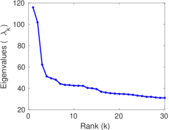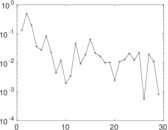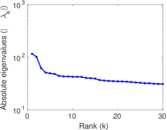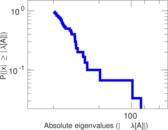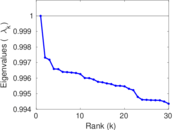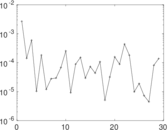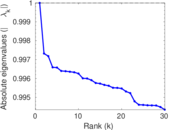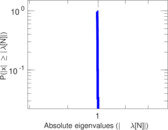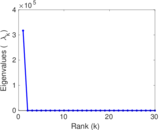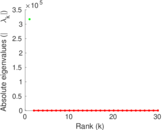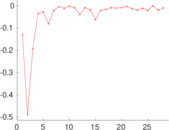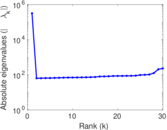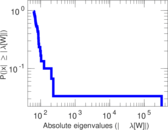# DBLP co-authorship

This is the co-authorship network of the DBLP computer science bibliography. Nodes are authors and an undirected edge between two nodes exists if the corresponding authors have published at least one paper together.

 Code `CD` Internal name `com-dblp` Name DBLP co-authorship Data source http://snap.stanford.edu/data/com-DBLP.html AvailabilityDataset is available for download Consistency checkDataset passed all tests Category Co-authorship network Node meaning Author Edge meaning Co-authorship Network format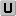Unipartite, undirected Edge typeUnweighted, no multiple edges LoopsDoes not contain loops Join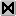Is the join of an underlying network

## Statistics

 Size n = 317,080 Volume m = 1,049,866 Loop count l = 0 Wedge count s = 21,780,889 Claw count z = 431,568,151 Cross count x = 11,548,309,777 Triangle count t = 2,224,385 Square count q = 55,107,655 4-Tour count T4 = 530,084,528 Maximum degree dmax = 343 Average degree d = 6.622 09 Fill p = 2.088 47 × 10−5 Size of LCC N = 317,080 Diameter δ = 23 50-Percentile effective diameter δ0.5 = 6.087 33 90-Percentile effective diameter δ0.9 = 8.164 68 Median distance δM = 7 Mean distance δm = 6.752 76 Gini coefficient G = 0.535 780 Balanced inequality ratio P = 0.298 185 Relative edge distribution entropy Her = 0.955 003 Power law exponent γ = 1.720 01 Tail power law exponent γt = 3.261 00 Tail power law exponent with p γ3 = 3.261 00 p-value p = 0.000 00 Degree assortativity ρ = +0.266 521 Degree assortativity p-value pρ = 0.000 00 Clustering coefficient c = 0.306 377 Spectral norm α = 115.847 Algebraic connectivity a = 0.012 818 8 Spectral separation |λ1[A] / λ2[A]| = 1.138 12 Non-bipartivity bA = 0.856 921 Normalized non-bipartivity bN = 0.032 705 7 Spectral bipartite frustration bK = 0.002 175 53

## Plots

### Fruchterman–Reingold graph drawing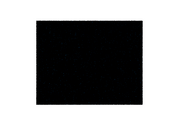### Degree distribution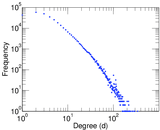### Cumulative degree distribution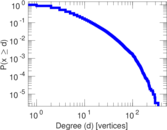### Lorenz curve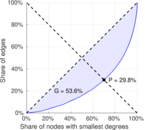### Spectral distribution of the adjacency matrix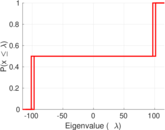### Spectral distribution of the normalized adjacency matrix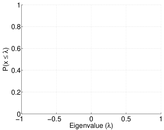### Spectral distribution of the Laplacian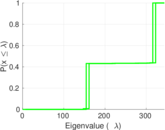### Spectral graph drawing based on the adjacency matrix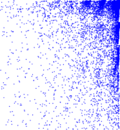### Spectral graph drawing based on the Laplacian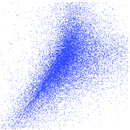### Spectral graph drawing based on the normalized adjacency matrix### Degree assortativity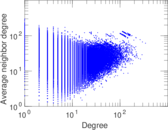### Zipf plot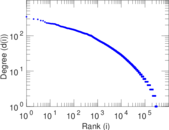### Hop distribution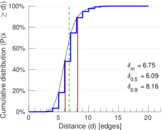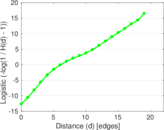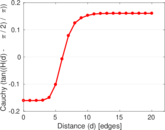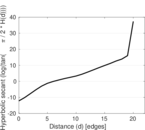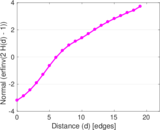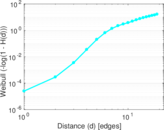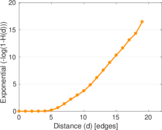### Delaunay graph drawing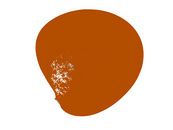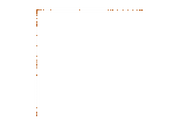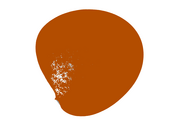### Clustering coefficient distribution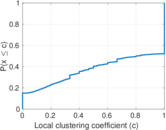### Matrix decompositions plots